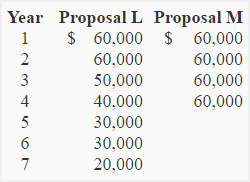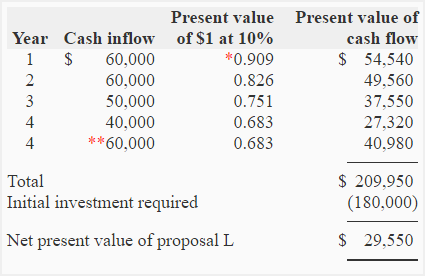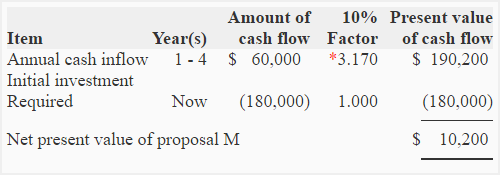# Exercise-6 (Capital budgeting with unequal proposal lives)

The National Food Company is comparing two proposals – proposal L and proposal M. Proposal L has a useful life of 7 years whereas proposal M has a useful life of 4 years. Both the proposals require an equal initial investment of \$180,000. The information about cash inflow expected from proposal L and proposal M is given below:The management of National Food Company wants a 10% rate of return on capital investments.

Required: Compare two proposals using net present value method. (Hint: An expert valuer estimates that the residual value of proposal L will be \$60,000 at the end of Year 4.)

## Solution:

### (1) Computation of net present value:

The two proposals have unequal useful lives and therefore their net present value cannot be directly compared. To make the proposals comparable, we assume that proposal L is terminated at the end of 4th year which is the end of the life of proposal M. For this purpose we have to estimate the residual value of proposal L and assume that the proposal is sold at its estimated residual value at the end of 4th year.

The net present value of proposal L and proposal M is computed below:

Net present value of proposal L:• *Value from present value of \$1 table.
• **Estimated residual value of the proposal L at the end of 4th year. The residual (or salvage) value estimated at any point of time might be different from the total expected future cash inflow to be generated by the proposal. In this exercise, the total expected cash inflow of years 5, 6 and 7 is \$80,000 (= \$30,000 + \$30,000 + \$20,000) but we have estimated a residual value of \$60,000 at the end of year 4. Such valuations are usually made by management or an independent expert valuer.

Net present value of proposal M:### (2) Decision:

Proposal L looks more desirable because its net present value is more than that of proposal M.

Show your love for us by sharing our contents.
A D V E R T I S E M E N T

### 4 Comments on Exercise-6 (Capital budgeting with unequal proposal lives)

1.priyal jain

i dnt understand ..y we had taken the value of 60k …in the proposal L..?

1.Accounting For Management

It is the estimated residual value.

2.K

Residual value of the proposal L assumed at the end of 4th year = 60,000

1.